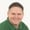FREELessons: 11Length: 46 minutes• Overview
• Transcript

# 2.1 Scalar Type Hints

PHP 5 introduced the idea of type hints, but support was limited to arrays and classes. In PHP 7, we finally have type hints for more primitive data types like int and string.

## 4.Conclusion1 lesson, 01:09

### 2.1 Scalar Type Hints

PHP 5 introduced type hints for complex data types like arrays and objects. But type hints for more primitive types like string, integer, float, and boolean were not supported. Well, we finally have type ints for those scalar types in PHP 7. And scalar type ints are just that, there are hints. It's a guide. It's not a strict type check. Instead it simply ensures that our function can work with the data that's passed to it. So let's write a function called sum. We have a and b as our parameters. And let's just echo the result of adding a and b together. So if we call the function passing one and two, we, of course, are going to see the value of three whenever our code executes. Now, let's change one of these parameters, it doesn't matter which one, to something that cannot be coerced into a number. Like a complete alpha string. So, if we try to run this code, we of course going to see a result. But it's the value of two. Which makes sense because our function Is adding two things together, a can't really be used so we end up with the value of b. Now, of course we could perform our own type checking inside of the function. But that's kind of why we have type-ins to begin with. So, now that we have scalar type ints, we can come in and we can say that a and b are integers. So if we pass something that cannot be coerced into an integer. Well we're going to run into an exception. We have a fatal error of the first argument has to some must be of type integer. Now it says that it must be of type integer but that's not really true. As long as it can be coerced into an integer. That's going to be fine. So if we pass in the string of one. Well, that is going to work. We once again have the value of three. And it works like this for all of our type ints. So let's pass in the string of one. Let's pass in a float 2.5. If we run this code we will once again get the value of three. So let's write another function. That's called simply output. And we'll look at a boolean value. And all we are going to do is simply dump the variable so that we can see the type and the value. So that's what we will see. And of course, if we pass in true or false we are going to see the results that we would expect. However, let's do output and then the integer of one. If we run this code we're going to see that it was coerced into a boolean value of true. If we pass in zero, then it's going to be a boolean value of false. And anything that is not zero is going to be true. And it helps if you save the file before you try to run it. Let's change our type int from bool to float. Let's pass in a string of 3.14. And whenever we run this in either the browser or whatever is running our code, we're going to see a float of 3.14. Now, if you want a strict type checking you can do that by setting a flag called strict types. So we're going to declare strict types equals one. And let's go back to the command line. Let's run the code and we see that we have an error. At the first argument has to output must be of type float. Now, in this case that is absolutely true. Because we are saying that our types are strict, you have to follow what we have. So in that case we have to pass in a floating point value. So that we can then execute our function. So we finally have scalar type ints with PHP 7. Frankly they should have been included with PHP five. But I guess later is better than never. And the wonderful thing is that we have several layers of strictness. We can have completely no strictness at all by just not using them. We can use type ints that give us a little bit of leeway. But if we want to be absolutely strict with our type ints we can set these strict types flag.

Back to the top